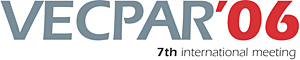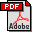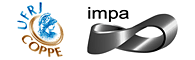vecpar.fe.up.pt/2006 | vecpar2006@fe.up.pt Block Iterative Algorithms for the Solution of Parabolic Optimal Control Problems Christian E. Schaerer (Instituto de Matematica Pura e Aplicada - IMPA)Tarek Mathew (Instituto de Matematica Pura e Aplicada - IMPA)Marcus Sarkis (Instituto de Matematica Pura e Aplicada - IMPA) Abstract: We consider block iterative methods for the solution of {\it large scale} linear-quadratic optimal control problems arising from the control of parabolic partial differential equations over a finite control horizon. This paradigm models new production strategies in oil and gas fields. To simulate the behavior in a reservoir under different scenarios, an optimal control problem can be formulated based on the constituent equations. After spatial discretization by finite element or finite difference methods, such problems typically require the optimal control of $n$ coupled ordinary differential equations, where $n$ can be quite large. Its solution by conventional methods can be prohibitively expensive in terms of computational cost and memory requirements. We describe two iterative algorithms. The first algorithm employs a CG method to solve a symmetric positive definite reduced linear system for the unknown control variable. A preconditioner is described, which we prove yields a rate of convergence independent of the space and time discretization parameters, however, double iteration is required. A second algorithm is designed to avoid double iteration by introducing an auxiliary variable. It yields a symmetric indefinite system, and for this system a positive definite block preconditioner is described. We prove a rate of convergence independent of the space and time discretization parameters when MINRES acceleration is used. Numerical results are presented for test problems. Keywords: Numerical Methods (Linear algebra), Numerical Methods (PDE), Parallel and Distributed Computing,Download the fullpaper TopRio de Janeiro | Brazil | 2006 | July | 10 11 12 13